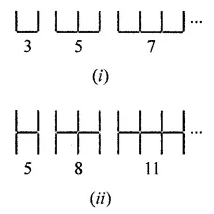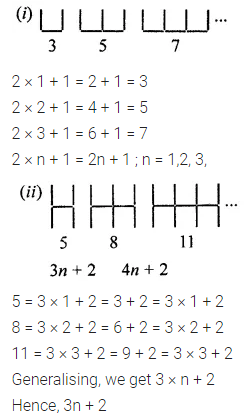# ML Aggarwal Class 7 Solutions for ICSE Maths Chapter 8 Algebraic Expressions Ex 8.4

## ML Aggarwal Class 7 Solutions for ICSE Maths Chapter 8 Algebraic Expressions Ex 8.4

Question 1.
Observe the patterns of shapes of letters formed from line segments of equal lengths:If n shapes of letters are formed, then write the algebraic expression for the number of line segments required for making these n shapes in each case.
Solution:Question 2.
Observe the pattern of shapes of digits formed from line segment of equal lengths:If n shapes of digits are formed, then write the algebraic expression for the number of line segments required for making these n shapes in each case.
Solution:Question 3.
If a pattern consists of n shapes formed by line segments of equal lengths and the number of line segments required to make n shapes is given by the algebraic expression:
(i) 2n + 1
(ii) 3n + 1
(iii) 3n + 2
(iv) 5n + 1
(v) 5n + 2
(vi) 4n + 3
then find the number of line segments required to form 5, 10 and 100 shapes in each case.
Solution: